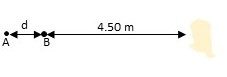Two small speakers A and B are driven in step at 725 Hz by the same audio oscillator. These...

Question:

Two small speakers A and B are driven in step at 725 Hz by the same audio oscillator. These speakers both start out 4.50 m from the listener, but speaker A is slowly moved away. The speed of sound in air is 344 m/s.

At what distance, d, will the sound from the speakers first produce destructive interference at the location of the listener?Destructive Interference

Destructive interference is defined as the superposition of the waves where they out phase each other and nullifies each other effect. Therefore there would be no sound when destructive interference takes place.

Given

Frequency {eq}(f) = 725 \ Hz {/eq}

Velocity of the wave {eq}(v) = 344 \ m/s {/eq}

Now, the wavelength is given by

{eq}\lambda = \dfrac{v}{f} \\ \lambda = \dfrac{344}{725} \\ \lambda = 0.47 \ m {/eq}

For the destructive interference path difference must be equal to the half of wavelength, hence

{eq}d = \dfrac{\lambda}{2} \\ d = \dfrac{0.47}{2} \\ d = 0.235 \ m {/eq}